# Waves

Also found in: Dictionary, Thesaurus, Medical, Financial, Acronyms, Idioms, Wikipedia.

## Waves

(Women Appointed for Voluntary Emergency Service), U.S. navy organization, created (1942) in World War II to release male naval personnel for sea duty. The organization was commanded until 1946 by Mildred Helen McAfee. Waves served in communications, air traffic control, naval air navigation, and clerical positions in the United States, Hawaii, Alaska, and the Caribbean. Recruiting ended in 1945, with a peak enrollment of 86,000. Waves forces were reduced when the war ended. After the passage (1948) of the Women's Armed Service Integration Act, women were enlisted into the regular navy, though they continued to be known as Waves for some time.

## Waves

changes in the state of a medium—disturbances— that propagate in the medium and carry energy with them. For example, an impact on the end of a steel rod produces local compression at that end, which then propagates along the rod at a velocity of about 5 km/sec; this is an elastic wave. Elastic waves occur in solids, liquids, and gases. Sound and seismic waves in the earth’s crust are special cases of elastic waves. Electromagnetic waves include radio waves, light, and X rays.

A fundamental property of all waves, regardless of their nature, is that in the form of a wave there is a transfer of energy without a transfer of matter (the latter can occur only as a side effect). For example, after a wave produced by a stone tossed into water has passed along the surface of the liquid, the particles of the liquid come to rest in approximately the same position as before the wave’s passage.

Wave processes are found in nearly all fields of physical phenomena; the study of waves is important for physics and engineering.

Waves may be classified according to the orientation of the disturbance with respect to the direction of their propagation. Thus, for example, a sound wave propagates through a gas in the same direction as the displacement of the gas particles (see Figure l,a); in a wave that is propagating along a string the points of the string are displaced in a direction perpendicular to the string (Figure 1,b). Waves of the first type are called longitudinal; of the second type, transverse.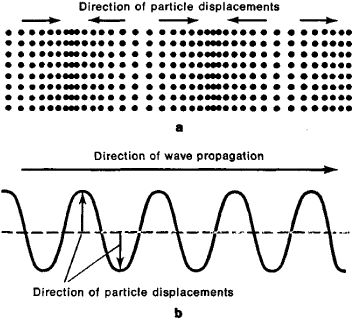Figure 1. (a) longitudinal wave, (b) transverse wave

Elastic forces develop in liquids and gases only upon compression and not in cases of shear; therefore, elastic deformations can propagate in liquids and gases only as longitudinal waves (“compression waves’). In solids, however, where elastic forces also develop in shear, elastic deformations can propagate both as transverse waves (“shear waves’) and longitudinal waves. In solid bodies of limited size—for example, rods and plates—the pattern of wave propagation is more complex, because in this case other types of waves arise that are combinations of the first two primary types.

In electromagnetic waves the direction of the electric and magnetic fields, except for certain cases of propagation in a restricted space, is almost always perpendicular to the direction of wave propagation; therefore, electromagnetic waves in free space are transverse.

General characteristics and properties. Waves may have various shapes. A relatively short disturbance that is irregular (see Figure 2,a) is called a single wave, or pulse. A limited series of repetitive disturbances is called a wave train. The concept of a train is usually applied to j section of a sine curve (Figure 2,b). The concept of the harmonic wave—that is, an endless sine wave in which all changes of the state of the medium follow the law of sines or cosines (Figure 2,c)—is of particular importance in wave theory; such waves, if their amplitude is small, can propagate in a homogeneous medium without form distortion (see below: Harmonic waves, Amplitude and phase). It is understood that the concept of an infinite sine wave is an abstraction that is applicable to a sine wave train of sufficient length.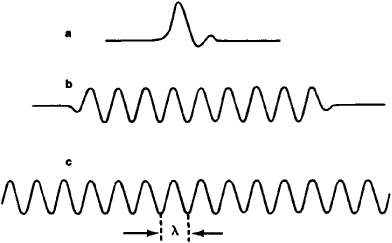Figure 2. (a) single wave, (b) wave train, (c) endless sine wave

The fundamental characteristics of harmonic waves are the wavelength λ, which is the distance between two maximums or minimums of the disturbance (for example, between adja-cent crests or valleys on the surface of water), and the period T, which is the time in which a particle of the medium completes one whole oscillation. Thus, an infinite wave has strict periodicity in space, which is observed, for example, in the case of elastic waves, even if only by means of instantaneous photography; and periodicity with respect to time, which is observed by following the motion of a given particle in the medium. A simple relationship exists between the wavelength λ and the period T. To obtain this relationship, a particle that is on a wave crest at a given moment is considered. After the first crest passes on, the particle falls into a trough, but after a certain time, equal to λ/c where c is the speed of propagation of the wave, a new crest will arrive, and the particle will again be on a crest as in the beginning. This process will be repeated regularly at time intervals equal to λ/c. The time λ/c coincides with the period T of the particle—that is, λ/c = T. This relationship is valid for harmonic waves of any kind.

The frequency v, which is equal to the number of periods per unit time (v = l/T), is often used instead of the period T. The relationship λv = c then exists between v and X. (In technology the symbol/is often used instead of v.} The concept of a wave vector having an absolute value k = 203V0;/λ = 2πv/c—that is, equal to the number of waves in the section 2π—and oriented in the direction of wave propagation is also used in wave theory.

Harmonic waves. Amplitude and phase. In a harmonic wave the changes in the oscillated quantity W with time occur according to the law of sines or cosines and are described at each point by the formula W = A sin 203C0;t/T. The value of W is assumed to be equal to zero for a state of equilibrium. A is the amplitude of the wave—that is, it is the value this quantity acquires upon the largest deviations from the state of equilibrium. At any other point located a distance r from the first point in the direction of propagation, the oscillations take place according to the same law but with a delay t1 = r/c, which can be written in the form

W = A sin(2π/T)(t - t1) = A sin(π/T)(t - r/c)

The expression Ø - (2π/T)(t - r/c) is called the phase of a wave. The phase difference between two points r1 and r2 is

Ø21-(2π/Tc)(r2-r1)=(2π/λ)(r2-r1)

At points separated from each other by an integral number of waves, the phase difference is the integer 2π—that is, oscillations at these points proceed synchronously, or in phase. On the other hand, at points separated from each other by an odd number of half-waves—that is, points for which r2-r1=(2N-1)λ/2, where N = 1,2,…—the phase difference is equal to an odd number of π:Ø21 =(2N-1)π The oscillations at such points proceed in opposite phase: at the moment when the deviation at one point is equal to A, it is of the opposite sign at the other point—that is, equal to —A—and vice versa.

Wave propagation is always associated with the transfer of energy, which may be characterized quantitatively by an energy flow vector I. For elastic waves this vector is called an Umov vector after the Russian scientist A. A. Umov, who introduced the concept, and for electromagnetic waves, a Poynting vector. The direction of an Umov vector is that of the energy transfer, and its absolute value is equal to the energy transferred by the wave per unit time through an area of 1 sq cm perpendicular to the vector I. For small deviations from an equilibrium state, I = KA, where A’ is a proportionality constant that depends on the nature of the wave and the properties of the medium in which it is propagating.

Equiphase surfaces and wave fronts. An important characteristic of a wave is the shape of its equiphase surfaces—that is, those surfaces for which at all points the phases are identical at a given moment. The shape of an equiphase surface is a function of the conditions of both the formation and propagation of a wave. In the simplest case such surfaces are planes that are perpendicular to the direction of wave propagation; the waves are called plane waves. Waves for which the equiphase surfaces are spheres and cylinders are called spherical and cylindrical waves respectively. The equiphase surfaces are also called wave fronts. In the case of a finite or a single wave the leading edge, which forms an immediate boundary between the wave and the undisturbed medium, is called the front.

Interference. When two waves arrive at a given point in a medium, their effect is additive. The superposition of so-called coherent waves—that is, waves having a constant phase difference at all times—is of particular significance. With coherent waves the phenomenon known as interference takes place: at the points where both waves arrive in phase, they reinforce each other; at points where they arrive in opposite phase, they weaken each other. As a result, a characteristic interference pattern is obtained.

Standing waves and natural oscillations. When a plane wave impinges on a plane reflecting obstacle, the reflected wave is plane. If there is no loss of energy as a wave propagates through a medium and is reflected from an obstacle, the amplitudes of the incident and reflected waves are equal. The reflected wave interferes with the incident wave, so that at those points where they are of opposite phase the resulting amplitude falls to zero—that is, the points remain at rest, form stationary oscillation nodes, and in the places where the wave phases coincide, reinforce each other, thus creating antinodes. The result is a so-called standing-wave pattern (see Figure 3). There is no energy flow in a standing wave: its energy, when there are no losses, only travels within the confines set by adjacent nodes and antinodes.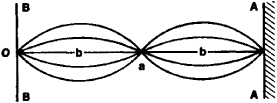Figure 3. Standing wave produced by the interference of waves incident on and reflected from an obstacle AA; point a is an oscillation node, and points b are antinodes

A standing wave can also exist in a bounded volume. In the particular case shown in Figure 3 one can imagine an obstacle at BB that is the same as the one on the right side. A standing wave will exist between the two walls if the distance between them is equal to an integral number of half-waves. Generally speaking, a standing wave can exist in a bounded volume only if the wavelength and the dimensions of the volume have a certain relationship. This condition is fulfilled for a series of frequencies v1,v2,v3, …, known as the natural frequencies of a given volume.

Diffraction. When a wave impinges on an opaque body or screen a shadow space is formed behind the body (the shaded area in Figure 4,a and 4,b). However, the boundaries of the shadow are diffuse rather than sharp, and the degree of diffusion increases with distance from the body. This phenomenon of the passage of waves around a body is called diffraction. At distances on the order of d2/λ from a body, where d is the body’s lateral dimension, the shadow is virtually completely blurred. The larger the body, the larger the space occupied by the shadow. Bodies whose dimensions are small compared with the wavelength do not create shadows at all but scatter the incident waves in all directions. The variation in the amplitude of a wave when passing from the“illuminated” region to the shadow region follows a complicated law, with alternating decrease and increase in amplitude (Figure 5) because of the interference of waves in rounding the body.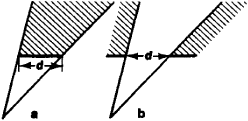Figure 4. Formation of a shadow when a wave is incident on (a) an opaque body and (b) an opening in an opaque screen (d is the dimension of the body or opening)

Diffraction also occurs when a wave passes through an opening (Figure 4,b), where it is also expressed in the penetration of waves into the shadow region and in some change in the character of the wave in the“illuminated” region: the smaller the diameter of the opening compared with a wavelength, the broader is the region into which the wave penetrates.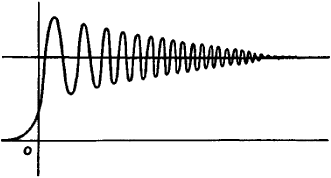Figure 5. Curve characterizing the illumination intensity of the space between light and shadow; the edge of the screen is at point O

Polarization. As has already been mentioned, the plane in which the oscillations in transverse waves occur is perpendicular to the direction of propagation. This feature of trans-verse waves makes possible the phenomenon of polarization, which consists of the disruption of the symmetrical distribution of the disturbances—for example, displacements and velocities in mechanical waves or electrical and magnetic field intensities in electromagnetic waves—relative to the direction of propagation. In a longitudinal wave, in which the disturbances are always oriented in the direction of the wave propagation, phenomena of polarization cannot occur.

If the oscillations E of a disturbance take place consistently in some single direction (Figure 6, a), the simplest case of a linearly polarized (plane-polarized) wave occurs. Other more complicated types of polarization are possible. For example,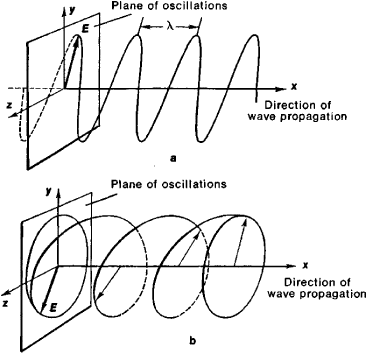Figure 6. (a) linearly polarized wave, (b) circularly polarized wave (E is a vector representing the propagating disturbance)

if the end of the vector E, which represents the disturbance, describes an ellipse or circle in the plane of the oscillations (Figure 6,b), elliptical or circular polarization occurs. The propagation velocity of transverse waves may depend on the state of polarization.

Polarization may occur because of a lack of symmetry in the radiator that excites the waves, because the wave is propagating through an anisotropic medium, or because of refraction and reflection of the wave at the interface of two mediums.

Reflection and refraction. When a plane wave is incident on a plane interface between two different mediums, it is partly reflected and partly transmitted into the other medium; it continues to be a plane wave, but it changes its direction of propagation—it is refracted (see Figure 7,a). The angles with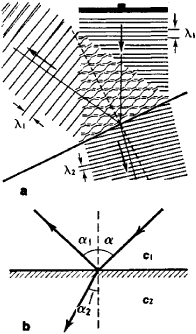Figure 7. (a) diagram of reflection and refraction for a plane wave (λ1, is the length of the incident and reflected waves; λ2 is the length of the refracted wave); (b) arrows representing rays corresponding to the incident, reflected, and refracted waves

the normal to the interface between the mediums formed by the directions of the incident, reflected, and refracted waves (Figure 7,b) are called the angle of incidence a, the angle of reflection α and the angle of refraction α2, respectively. According to the law of reflection, the angle of incidence equals the angle of reflection—that is, α=α1 According to the law of refraction, the sine of the angle of incidence is related to the sine of the angle of refraction as the wave velocity in the first medium to the velocity in the second medium—that is:

sin α/sin α2=c1/c2 = n

where n is the index of refraction.

A mixture of waves with different states of polarization and propagating in the same direction separates when it enters a medium for which the velocity of propagation is a function of the state of polarization; waves that are polarized differently will go in different directions (double refraction). In many cases the velocity of propagation is also a function of the frequency of the oscillations (dispersion); in such cases a mixture of waves of various frequencies is sepa-rated by refraction. Certain peculiarities develop during the reflection of diverging spherical or cylindrical waves at small angles to the plane of the interface between two mediums.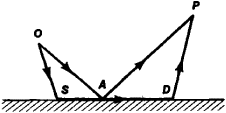Figure 8. Diagram of the formation of a side wave

The most important occurs when the wave velocity c2 in the lower medium is greater than the velocity c1 in the upper medium (Figure 8), in which case a so-called side wave ap-pears in addition to the usual reflected wave corresponding to the ray OAP. The ray OSDP, which corresponds to the side wave, passes in part (section SD) through the medium from which it is being reflected.

Waveform. Dispersion and nonlinearity of waves. During the process of propagation, the shape of a wave undergoes changes. The nature of the changes depends essentially on the initial shape of the wave. Only an infinite sine (harmonic) wave, except for waves of very high intensity, maintains its shape unchanged as it propagates, provided that it does not undergo appreciable absorption. However, all waves (of any shape) may be represented as the sum of infinite sine waves of various frequencies (that is, they may be decomposed into their spectra). For example, a single pulse may be represented as the infinite sum of sine waves that are superim-posed on each other. If the medium in which a wave is propagating is linear—that is, if its properties do not change under the influence of the disturbances created by the wave—then all the effects produced by nonharmonic waves may be defined as the sum of the effects produced separately by each of the wave’s harmonic components (the so-called principle of superposition).

In real mediums the propagation velocity of sine waves is often frequency-dependent (so-called wave dispersion). Consequently a nonharmonic wave—that is, a set of harmonic waves of various frequencies—changes its shape as it propa-gates, because during the propagation of these harmonic waves the relationships among their phases change. The wave form may also be distorted upon diffraction or scattering of nonharmonic waves, since both of these processes are dependent on wavelength, and therefore the harmonics of various wavelengths are affected differently. When dispersion is present, a change in the form of a nonharmonic wave may also occur as a result of refraction. However, the form of a harmonic wave may sometimes also be distorted. This happens when the amplitude of a propagating wave is so large that it is impossible to neglect the changes in the properties of the medium because of wave action; that is, when the non-linear properties of the medium exert an effect. The distortions of a sine wave may be manifested in the fact that the“humps” of the wave (the regions of greatest disturbance) propagate at a higher velocity than the other portions of the wave, thus resulting in the transformation of a sinusoidal shape into a sawtooth. In a nonlinear medium other laws of wave propagation are altered considerably, particularly the laws of reflection and refraction.

Phase and group velocities. The wave velocity introduced above, called the phase velocity, is the velocity at which any specified phase of an infinite sine wave, such as the phase corresponding to a crest or valley, is moving. The phase velocity of a wave, in particular, is part of the formula for the law of refraction. However, in practice the main concern is with finite waves rather than with waves that are in the form of infinite sinusoids (also known as monochromatic waves), for which the concept of phase velocity alone has meaning. As has already been indicated, any finite wave may be rep-resented as the superposition of a large number (more accurately, an infinitely large number) of monochromatic waves of various frequencies. If the phase velocities of the waves are the same for all frequencies, then the entire set or group of waves propagates with this velocity. If, however, these velocities are not identical—that is, if there is dispersion— the problem of the propagation velocity for a finite wave becomes complicated. The English physicist J. W. Rayleigh showed that if a finite wave is composed of waves having frequencies that differ little from each other, then this wave or, as it is often called, wave packet, propagates with a certain velocity, called the group velocity. The group velocity u is calculated according to the formula u = c - λdc/dλ. An energy transfer also takes place with the group velocity of the wave.

Change of frequency of a wave upon motion of source or observer (Doppler effect). An observer who is moving toward a source of waves of any kind perceives a certain increase in the frequency by comparison with an immobile observer, and an observer who is moving away from the source perceives a reduction in the frequency. A qualitatively similar phenomenon also occurs when an observer is immobile and the source of waves moves. This phenomenon is called the Doppler effect.

Waves and rays. A line that is oriented at each point in the direction of energy flow in a wave is called a ray (Figure 7,b). In an isotropic medium this direction coincides with the normal to the wave front. A parallel packet of straight lines corresponds to a plane wave, a packet of radially diverging lines corresponds to a spherical wave, and so on. Under certain conditions a complicated wave-propagation calculation can be replaced by a simpler calculation of the shape of the rays. This is used in geometric acoustics and geometric optics. This simplified approach is valid when the wavelength is small enough in comparison to certain characteristic dimensions—for example, the dimensions of obstacles lying in the path of propagation of a wave, the transverse dimensions of the wave front, and the distances from the points at which the waves converge.

Radiation and propagation. In order for the radiation of waves to occur, a disturbance must be produced in a medium by an external source of energy. The work performed by this source, less certain losses, is converted into the energy of the radiated waves. Thus, for example, the diaphragm of a tele-phone or loudspeaker, upon receiving energy from an electroacoustic transducer, radiates sound waves. Waves are always radiated from sources of limited dimensions; as a result,“diverging” waves arise. These waves may be regarded as plane waves only at a sufficiently great distance from the source.

Despite the various natures of waves, the principles that govern their propagation have much in common. Thus, elastic waves in homogeneous fluids (gases) or electromagnetic waves in free space (and in certain cases in a space filled with a homogeneous isotropic dielectric) that are produced in any small region (a“point”) and which propagate without absorption into the surrounding space are subject to the same wave equation.

A special kind of wave radiation occurs during the motion of bodies in a medium with velocities greater than the phase velocities of waves in the medium. An electron that is moving in any medium at a velocity greater than the phase velocity of electromagnetic waves in the medium radiates waves (Cherenkov-Vavilov radiation), but when moving at a velocity less than the phase velocity of light in the medium, this motion is accompanied only by a simple displacement of the electrical and magnetic fields, without transferring the energy of motion into the energy of radiation. Similarly, an airplane moving at a speed greater than the speed of sound radiates a sound wave of a special kind—a shock wave—and gives up a certain portion of its energy to the wave. The radiation of waves of the same origin propagating along the surface of water explains the appearance of wave drag during the movement of a ship.

Other kinds of waves. Other kinds of waves include temperature waves, which propagate in the vicinity of a heat source that is varying with time; viscosity waves, which are rapidly attenuated transverse waves in a viscous liquid; de Broglie waves, which are used to describe the behavior of elementary particles in quantum mechanics; and gravitational waves, which are radiated by masses that are moving with acceleration.

### REFERENCES

Gorelik, G. S. Kolebaniia i volny, 2nd ed. Moscow, 1959.
Krasil’nikov, V. A. Zvukovye i ul’trazvukovye volny v vozdukhe, vode i tverdykh telakh, 3rd ed. Moscow, 1960.
Brekhovskikh, L. M. Volny v sloistykh sredakh. Moscow, 1957.

L. M. BREKHOVSKIKH

## What does it mean when you dream about waves?

Water is a common symbol for the emotions. Waves, being either smooth or violent, may therefore indicate either emotional calm or emotional turbulence.

References in classic literature ?
He rose on the waves, making signs of distress; but no one on board saw him, and the vessel stood on another tack.
He saw overhead a black and tempestuous sky, across which the wind was driving clouds that occasionally suffered a twinkling star to appear; before him was the vast expanse of waters, sombre and terrible, whose waves foamed and roared as if before the approach of a storm.
Often in prison Faria had said to him, when he saw him idle and inactive, "Dantes, you must not give way to this listlessness; you will be drowned if you seek to escape, and your strength has not been properly exercised and prepared for exertion." These words rang in Dantes' ears, even beneath the waves; he hastened to cleave his way through them to see if he had not lost his strength.
The coop did not toss around any more, but rode the waves more gently--almost like a cradle rocking--so that the floor upon which Dorothy stood was no longer swept by water coming through the slats.
"Why, I've got a ship of my own!" she thought, more amused than frightened at her sudden change of condition; and then, as the coop climbed up to the top of a big wave, she looked eagerly around for the ship from which she had been blown.
Seeing in what great distress Ulysses now was, she had compassion upon him, and, rising like a sea-gull from the waves, took her seat upon the raft.
He swam seaward again, beyond reach of the surf that was beating against the land, and at the same time he kept looking towards the shore to see if he could find some haven, or a spit that should take the waves aslant.
Indeed, the volume of water in the Mediterranean, incessantly added to by the waves of the Atlantic and by rivers falling into it, would each year raise the level of this sea, for its evaporation is not sufficient to restore the equilibrium.
With the sun rose the Fairies, and, with Eva, hastened away to the fountain, whose cool waters were soon filled with little forms, and the air ringing with happy voices, as the Elves floated in the blue waves among the fair white lilies, or sat on the green moss, smoothing their bright locks, and wearing fresh garlands of dewy flowers.
Beneath green arches, bright with birds and flowers, beside singing waves, went Eva into a lofty hall.
"Assuredly I do; I would willingly risk and lose my life in an encounter against men," he said, glancing at Bragelonne, "but as to fighting with oars against waves, I have no taste for that."
"Courage, my men," said De Guiche; "I have twenty pistoles left in this purse, and as soon as we reach the admiral's vessel they shall be yours." The sailors bent themselves to their oars, and the boat bounded over the crest of the waves. The interest taken in this hazardous expedition was universal; the whole population of Havre hurried towards the jetties and every look was directed towards the little bark; at one moment it flew suspended on the crest of the foaming waves, then suddenly glided downwards towards the bottom of a raging abyss, where it seemed utterly lost.

Site: Follow: Share:
Open / Close